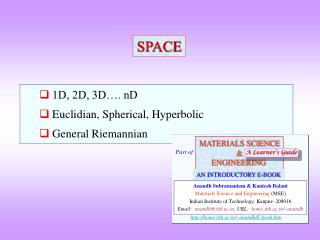DownloadDownload Presentation1D, 2D, 3D…. nD Euclidian, Spherical, Hyperbolic General Riemannian

# 1D, 2D, 3D…. nD Euclidian, Spherical, Hyperbolic General Riemannian

Télécharger la présentation## 1D, 2D, 3D…. nD Euclidian, Spherical, Hyperbolic General Riemannian

- - - - - - - - - - - - - - - - - - - - - - - - - - - E N D - - - - - - - - - - - - - - - - - - - - - - - - - - -
##### Presentation Transcript

1. MATERIALS SCIENCE & ENGINEERING Part of A Learner’s Guide AN INTRODUCTORY E-BOOK Anandh Subramaniam & Kantesh Balani Materials Science and Engineering (MSE) Indian Institute of Technology, Kanpur- 208016 Email:anandh@iitk.ac.in, URL:home.iitk.ac.in/~anandh http://home.iitk.ac.in/~anandh/E-book.htm SPACE 1D, 2D, 3D…. nD Euclidian, Spherical, Hyperbolic General Riemannian

2. SPACE • A simplistic point of view is that space is a perfect inert vacuum and matter resides in that space. • The real picture is more complicated, with actual space deviating from the ‘smooth picture’ at the very small lengthscales. Space at these lengthscales is teeming with virtual particles which fleetingly come into existence. • There are unsolved questions regarding the number of dimensions and curvature of space we live in → though it is assumed to be 3D Euclidean locally* → At the scale of the universe it is understood to be non-Euclidean • Many of the theories of physics which describe nature (e.g. the string theories) require higher dimensions (10 or more in some of them!). Dimensions higher than 3 are supposed to be ‘compactified’ • Apart from the possibility of higher spatial dimensions, in Einstein’s description of gravity, the 3D space is intertwined with time into a 4D ‘space-time unit’. • In some theories, the structure of glasses and quasicrystals are described by hyper-dimensional constructs. * refer next slide

3. SPACE continued… • Gaussian curvature is the product of two orthogonal curvatures • Mean curvature is the average of two orthogonal curvatures • Space can be:Euclidean (flat) → Zero (0) Gaussian Curvature  Spherical → Positive (+) Gaussian Curvature  Hyperbolic → Negative () Gaussian Curvature • If for a Hyperbolic surface the mean curvature is zero then the surface is called a Minimal Surface Emphasis though it is assumed that space is: 3D → in general it can be nD Euclidean → it can be Non-Euclidean with local variations in the curvature of space